# Nuances of Hose Making

2021/07/04

Here's a polyline: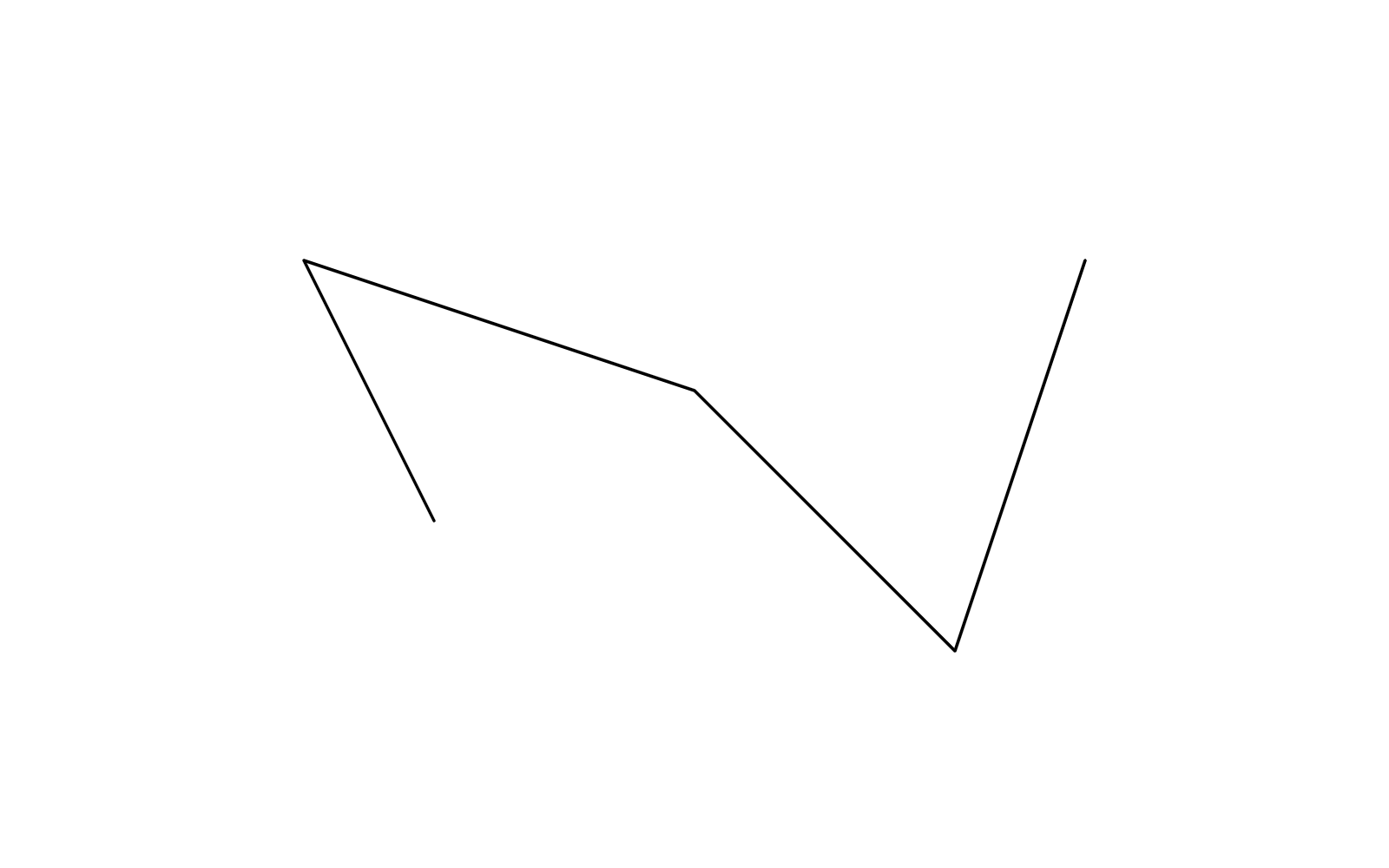You want to make it thicker. Being a lazy person, you just draw a rotated rectangle for each segment: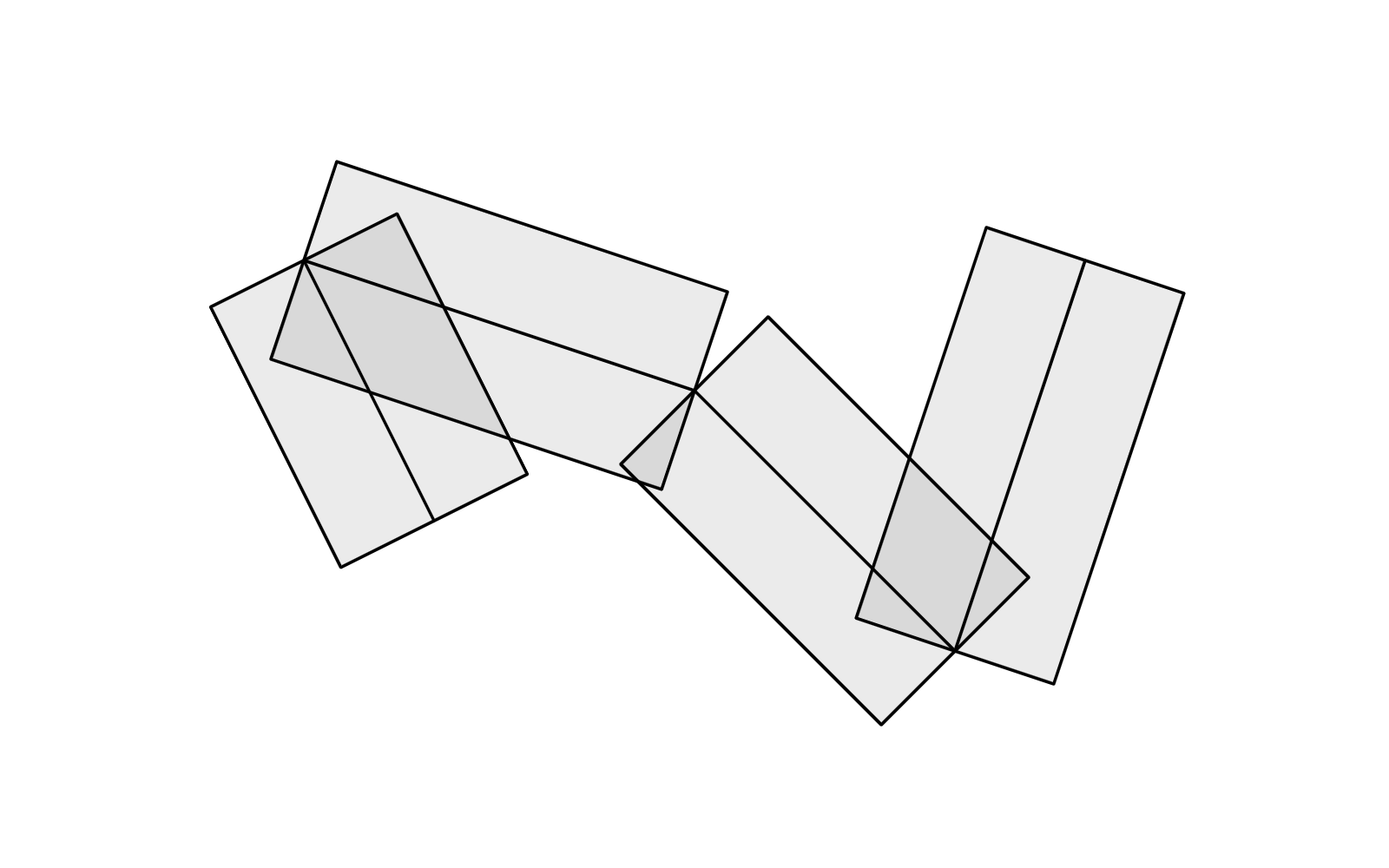Uhm… the joints are kind of ugly. No problem! Stick a circle to each:Solved. But what if we want to find the outline? Some sort of union of the rects and circles. Something like this:That's a bit trickier, we'll cover how to do that later in this article. But for now, there's another question: What if we want variable thickness at each polyline vertex?

Easy, you might think. Just change the radius of each circle, and turn the rectangles to trapezoids. Like this: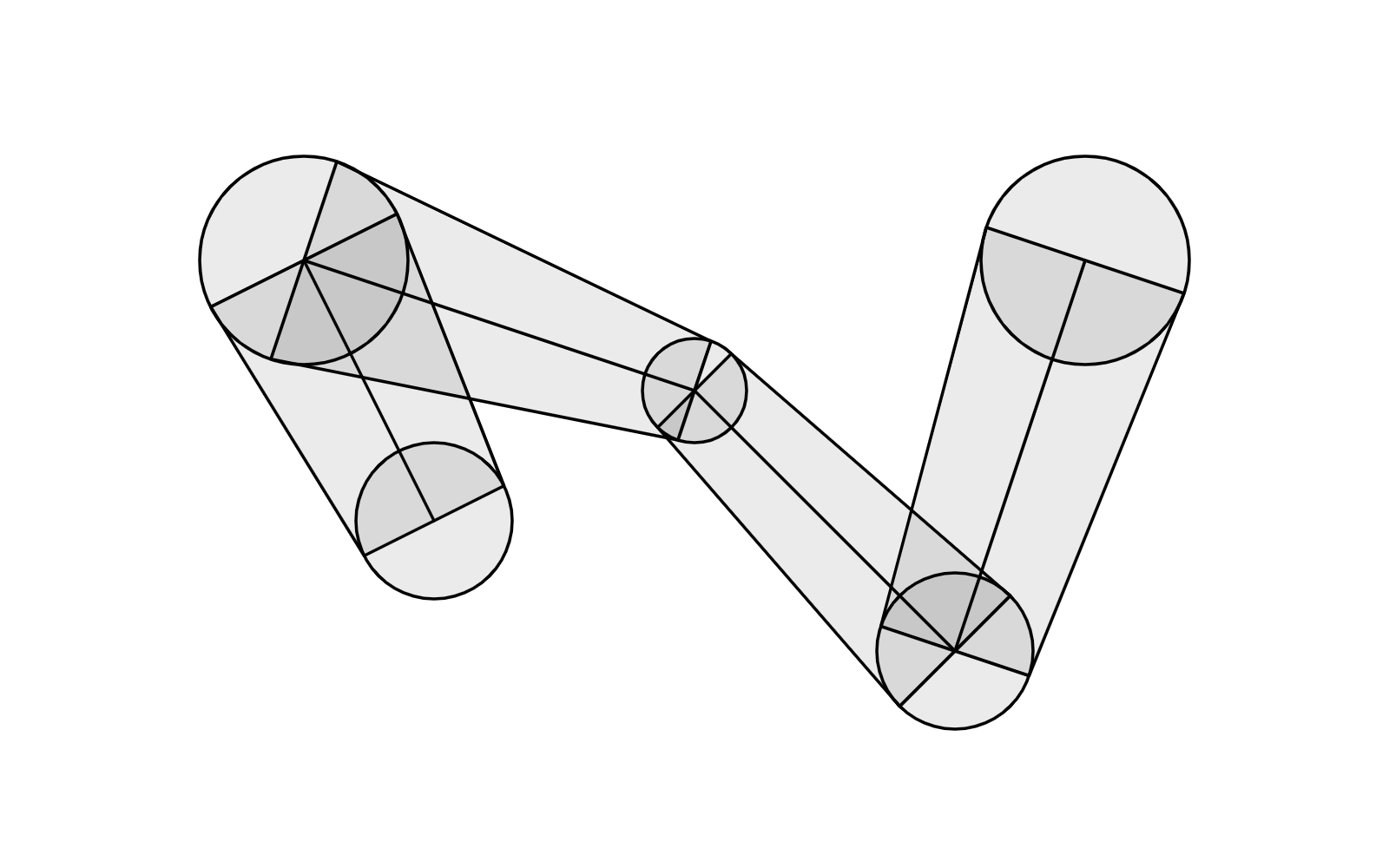Looks alright… until you try to increase the variance of the radii. Now you'll start to see the problem: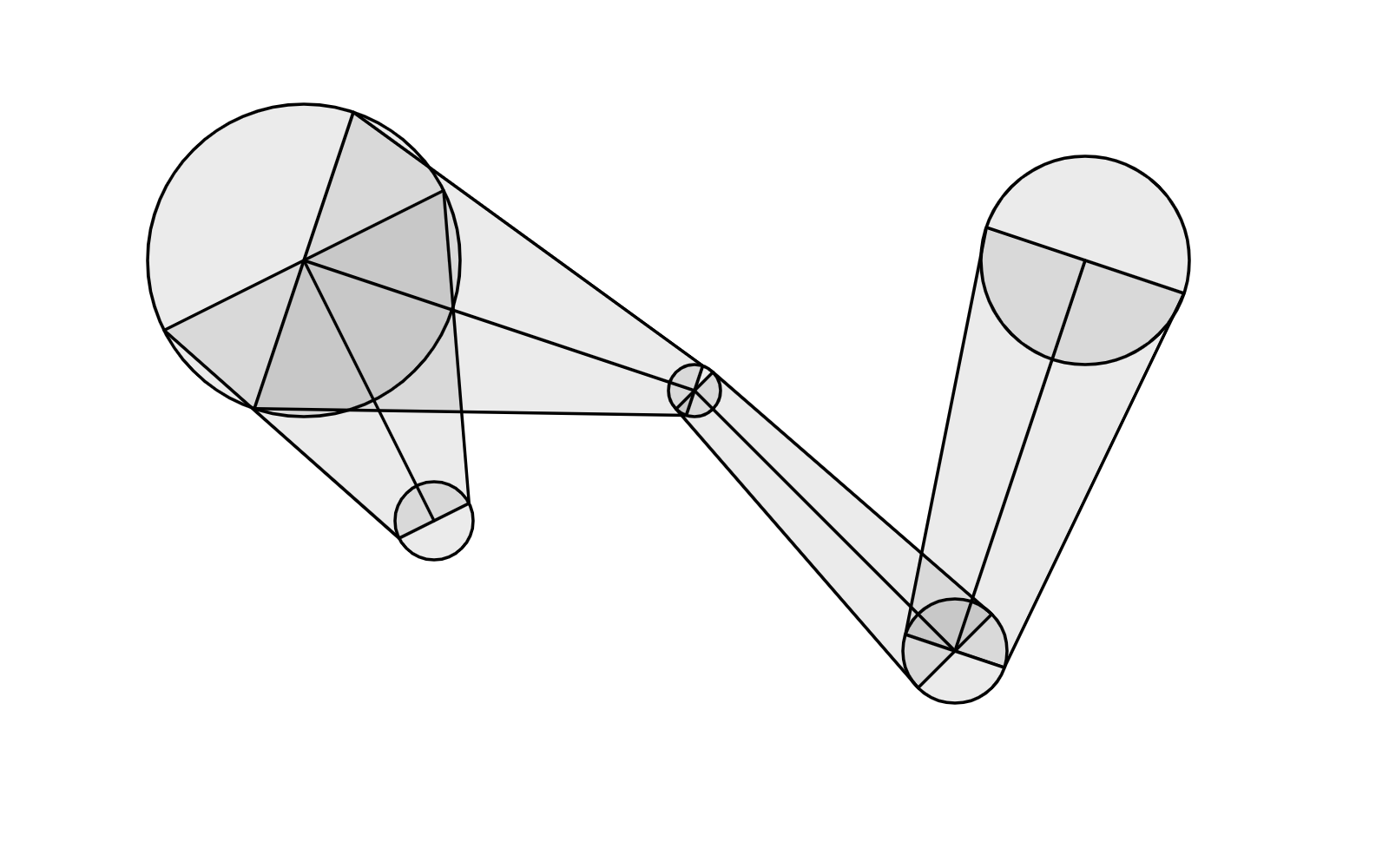The problem is even more obvious when you look at the outline: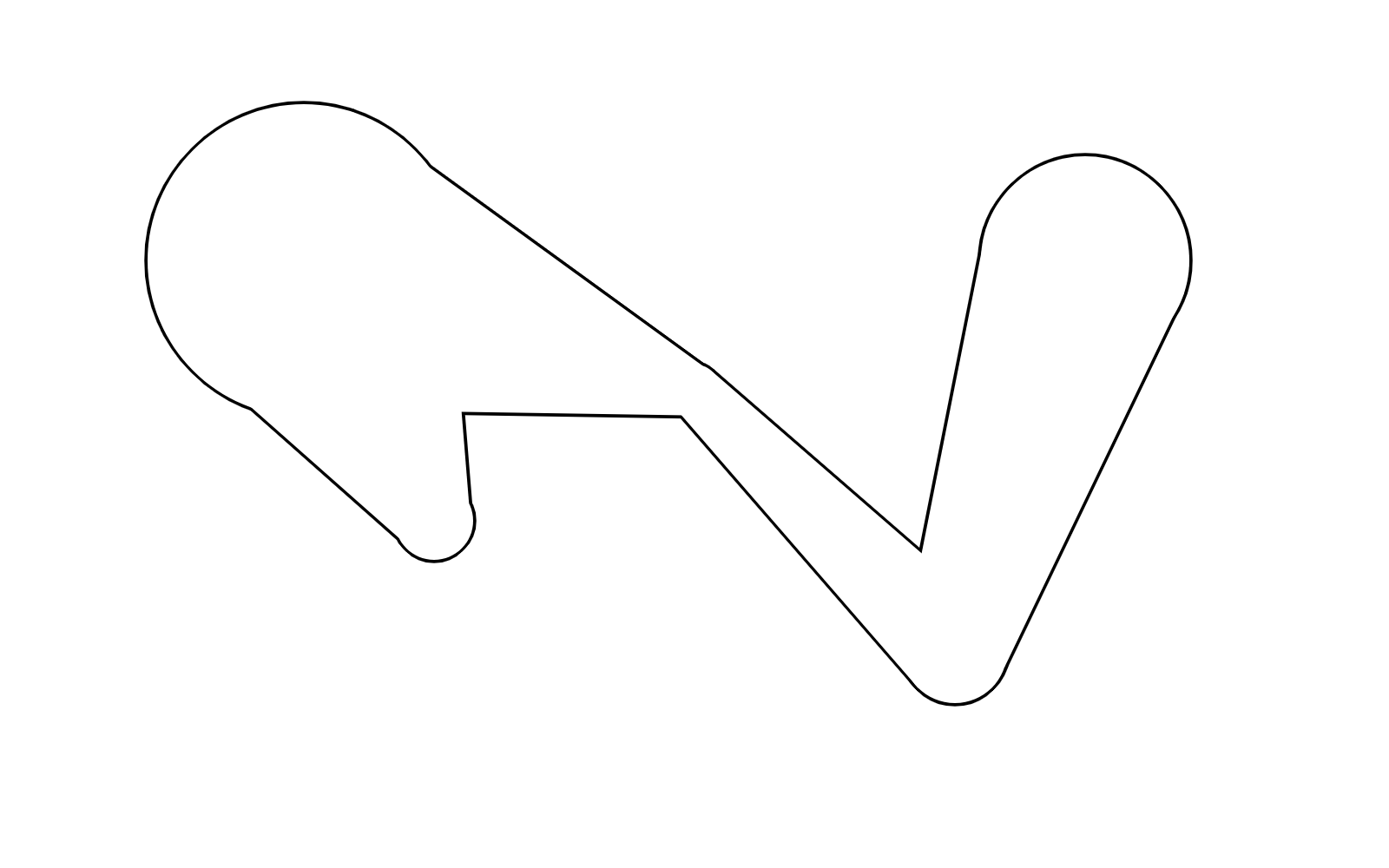Ew! Look at those bumps! What you actually want is something smoother, like this: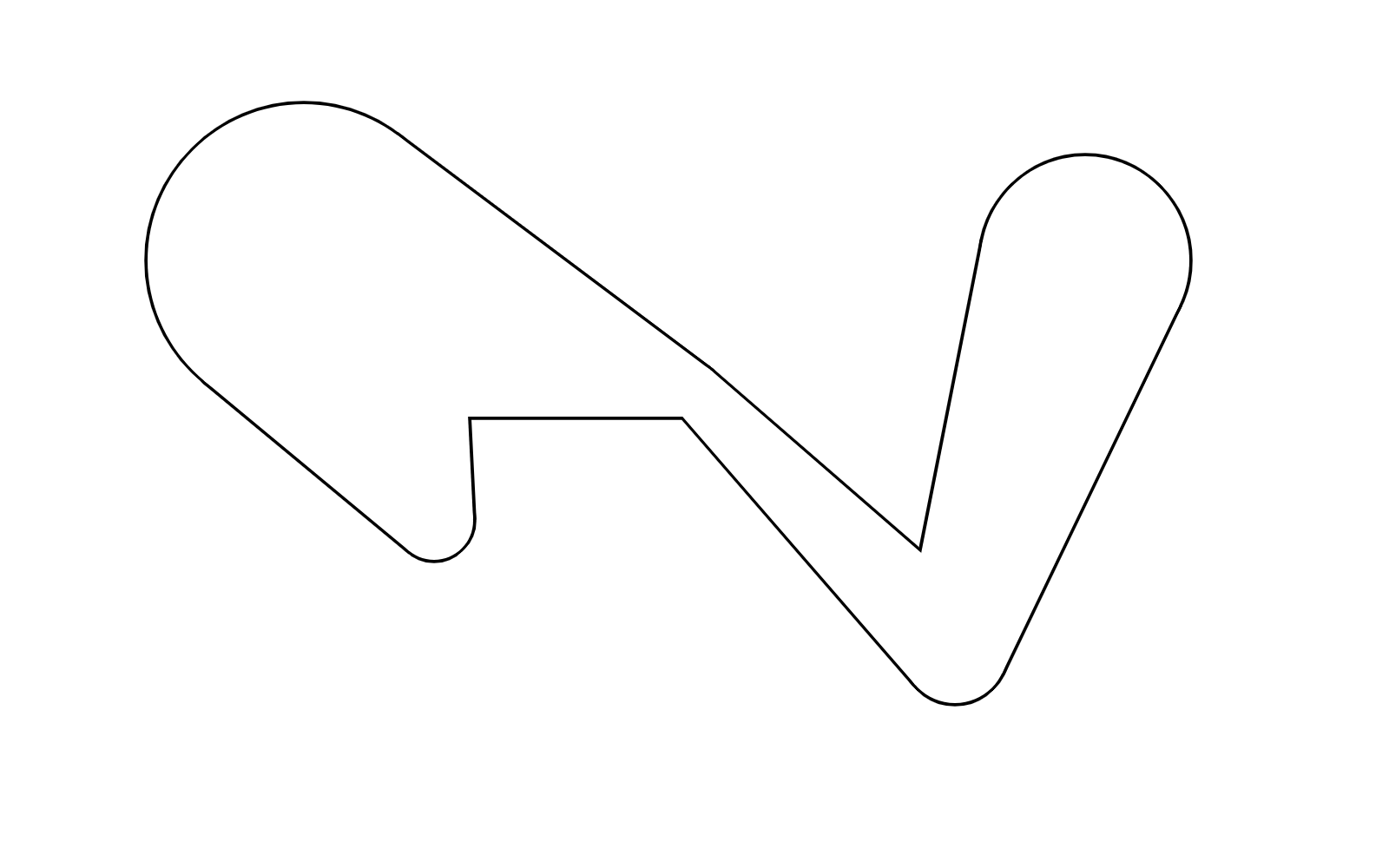What's the problem? Here's the problem: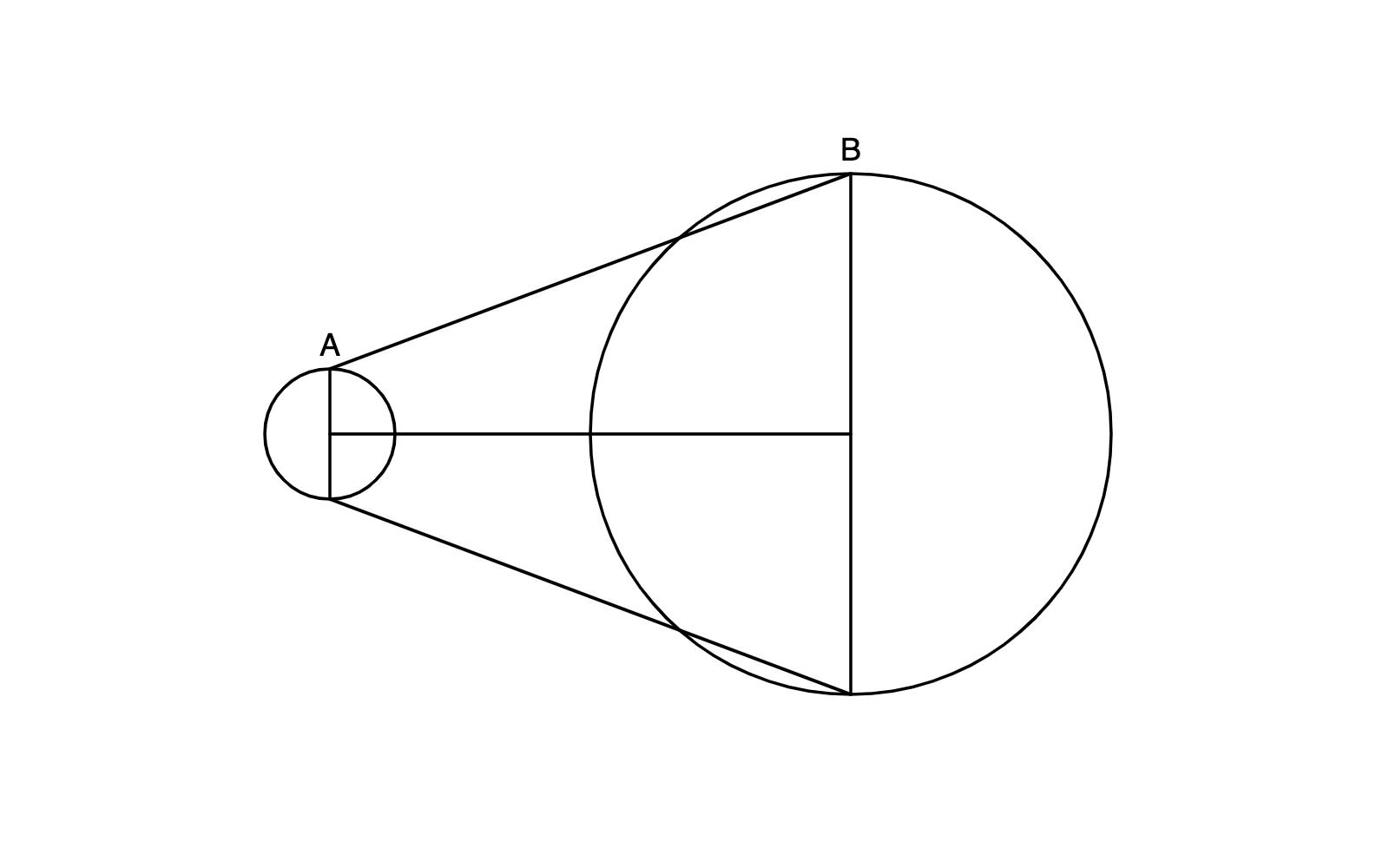We want to find a line that is tangent to both the small circle and the large circle. The $AB$ in the diagram above is NOT the line we want.

Here's how to find the correct $AB$: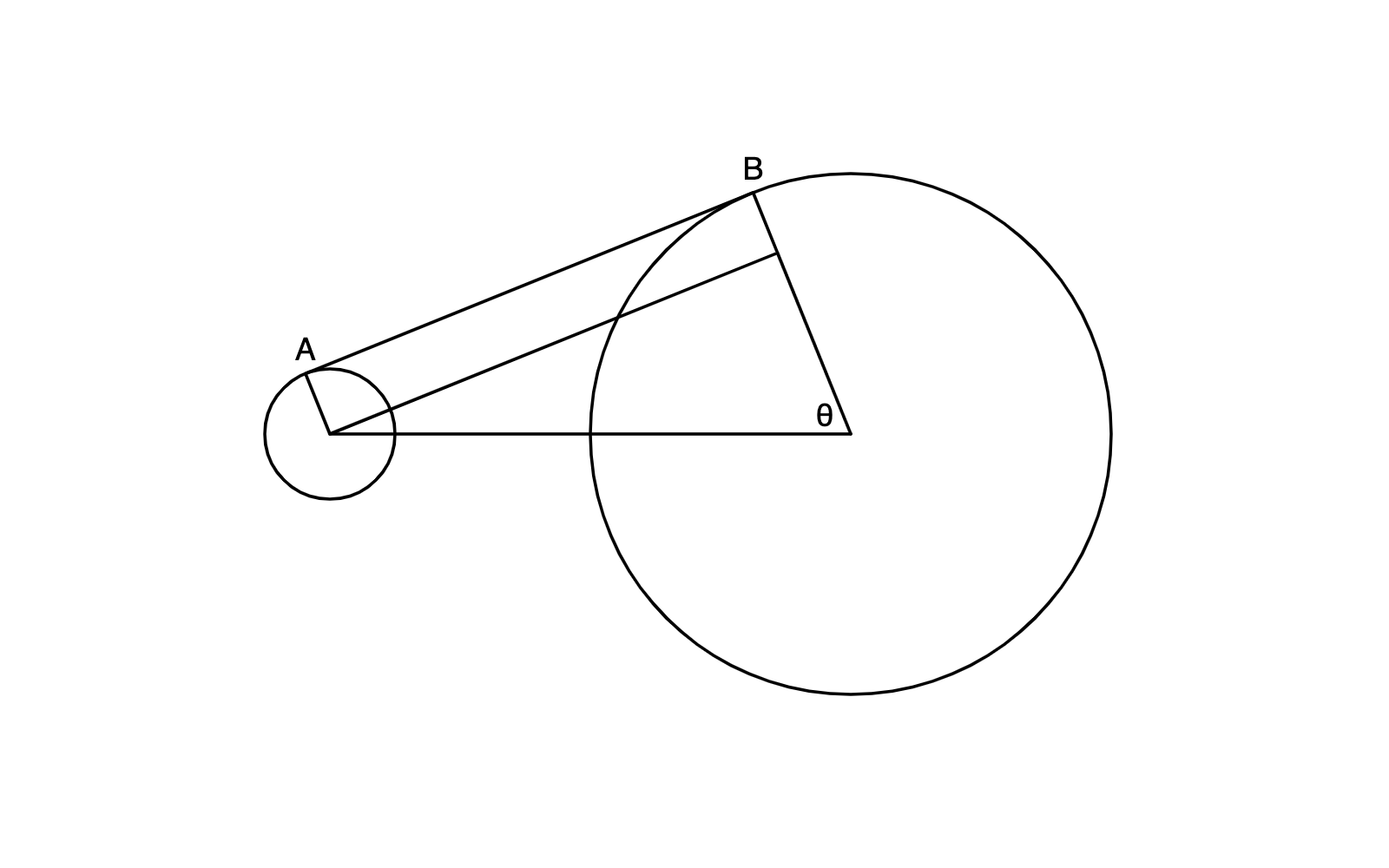The key is to find the angle $\theta$. If the radii are equal, we get lucky and $\theta=90\degree$ and our rectangle-drawing will work. Otherwise we need to actually find theta:

• Let $r$ be the radius of the small circle, and $R$ be the radius of the larger circle.
• Let $d$ be the distance between the center of the circles.
• $\theta=\arccos(\frac{R-r}{d})$

We do this computation for every vertex on the polyline: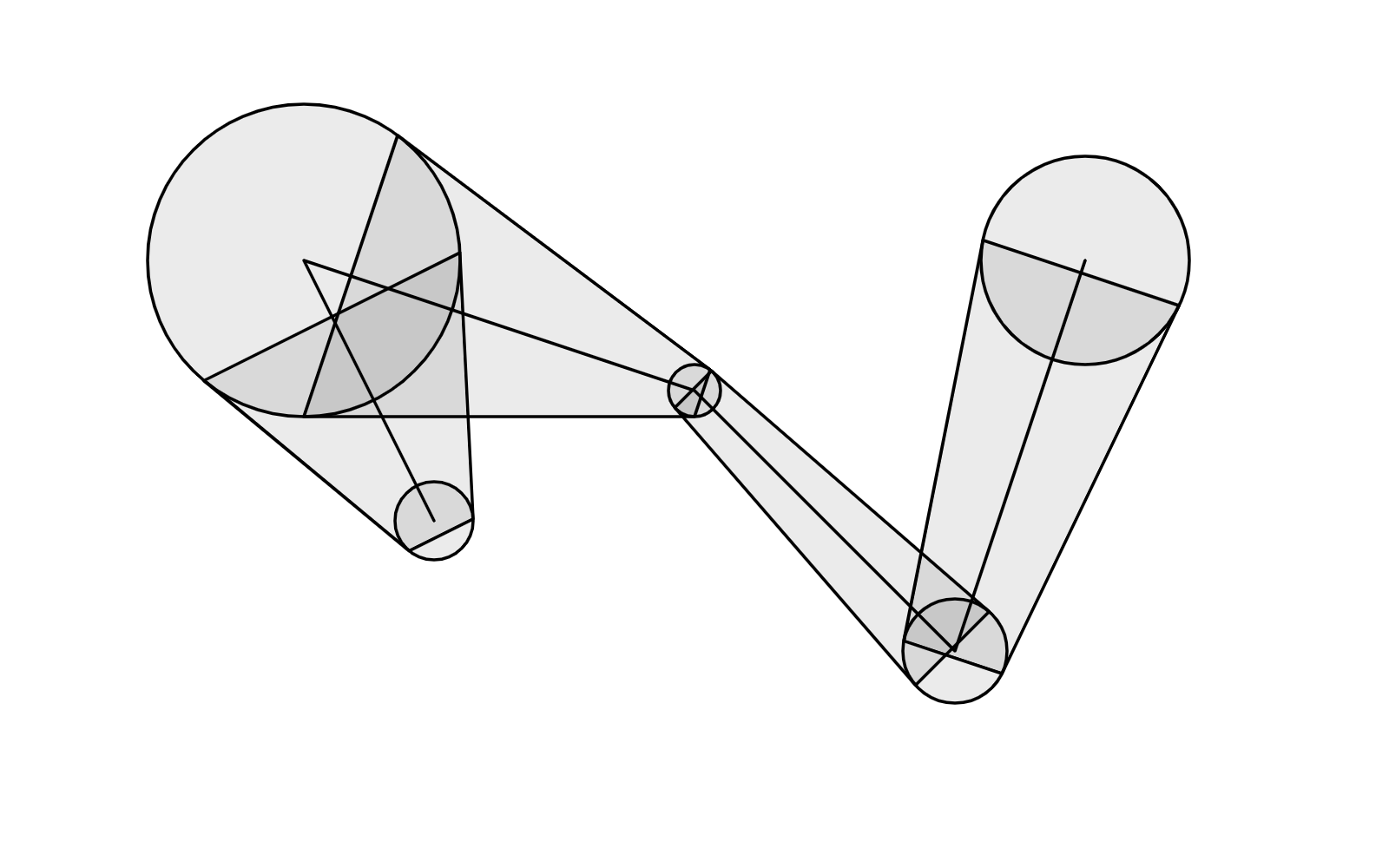Ahh! Nice.

## Finding the Outline

Now we find the outline.

For each edge, we find the four corners of the trapezoid. We store the corners on the right side of each edge (red dots) in one array, and those on the left (blue dots) in another array.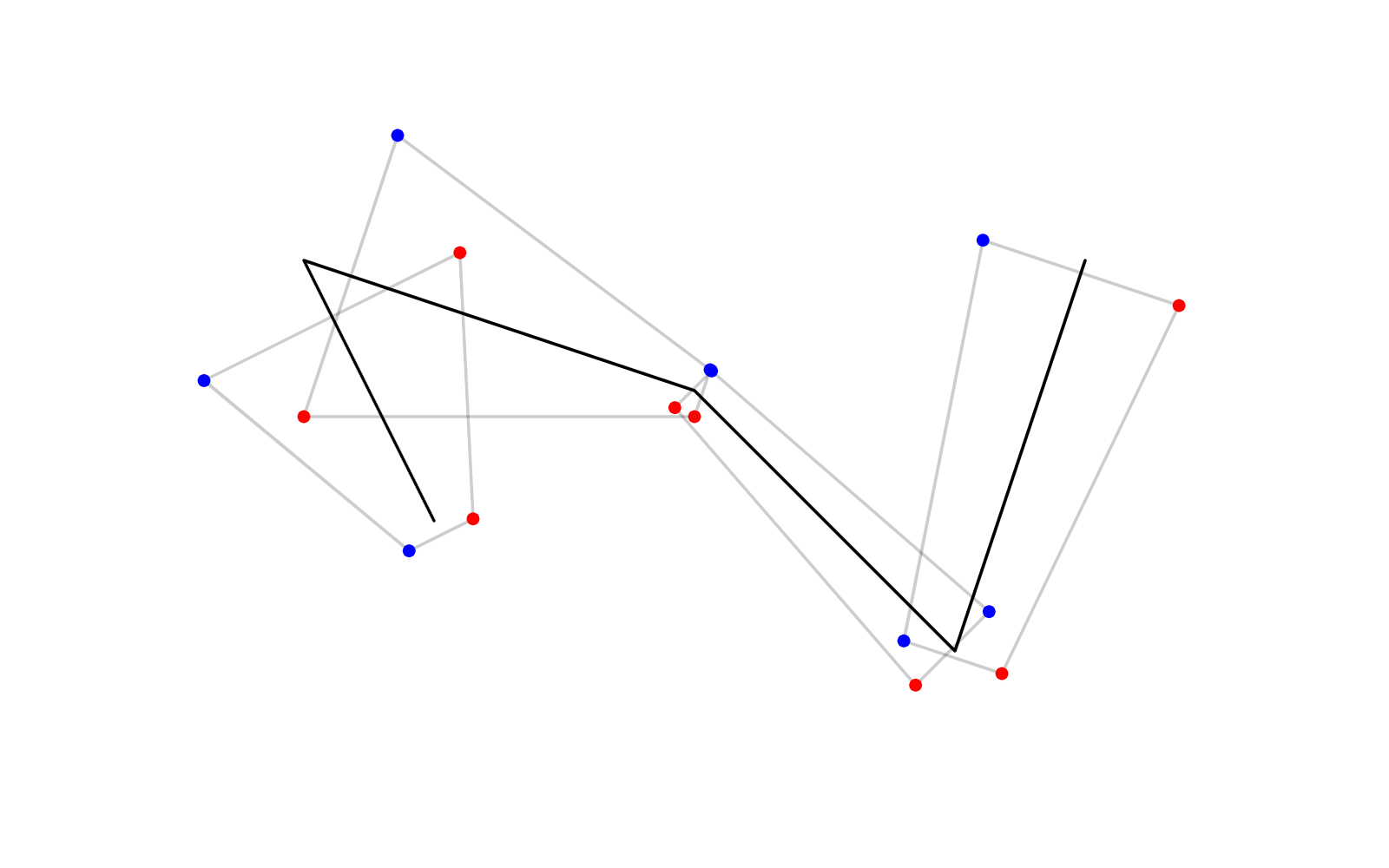We can connect the dots in the blue array and the dots in the (reversed) red array to generate an outline. We don't have the rounded joints yet, and this is usually referred to as “bevel” join style.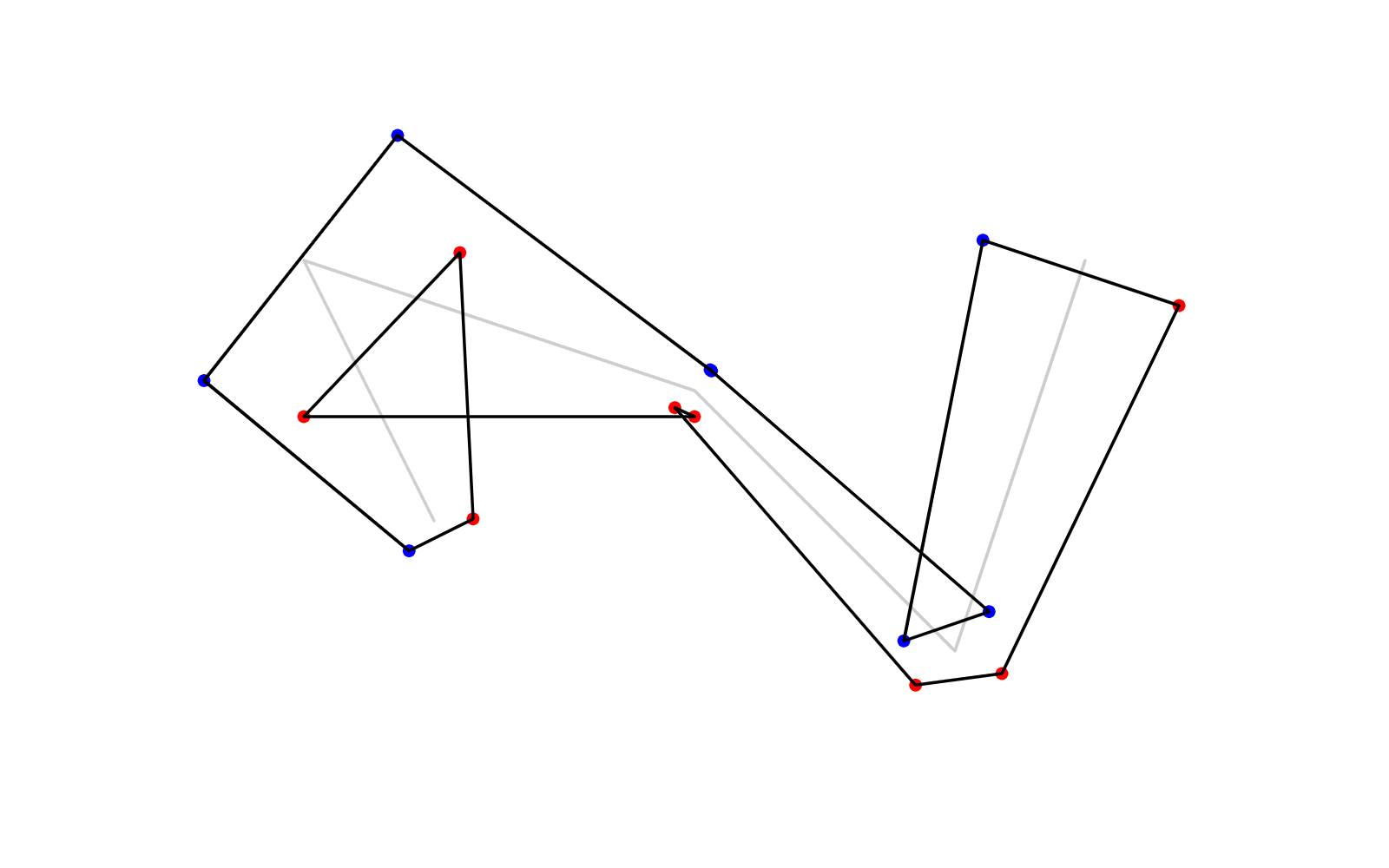Now we figure out how to add the round joints. In the general case, if the polyline turns left at a vertex, we need to add an arc to the right side; if the polyline turns right at a vertex, we need to add an arc to the left side, like so: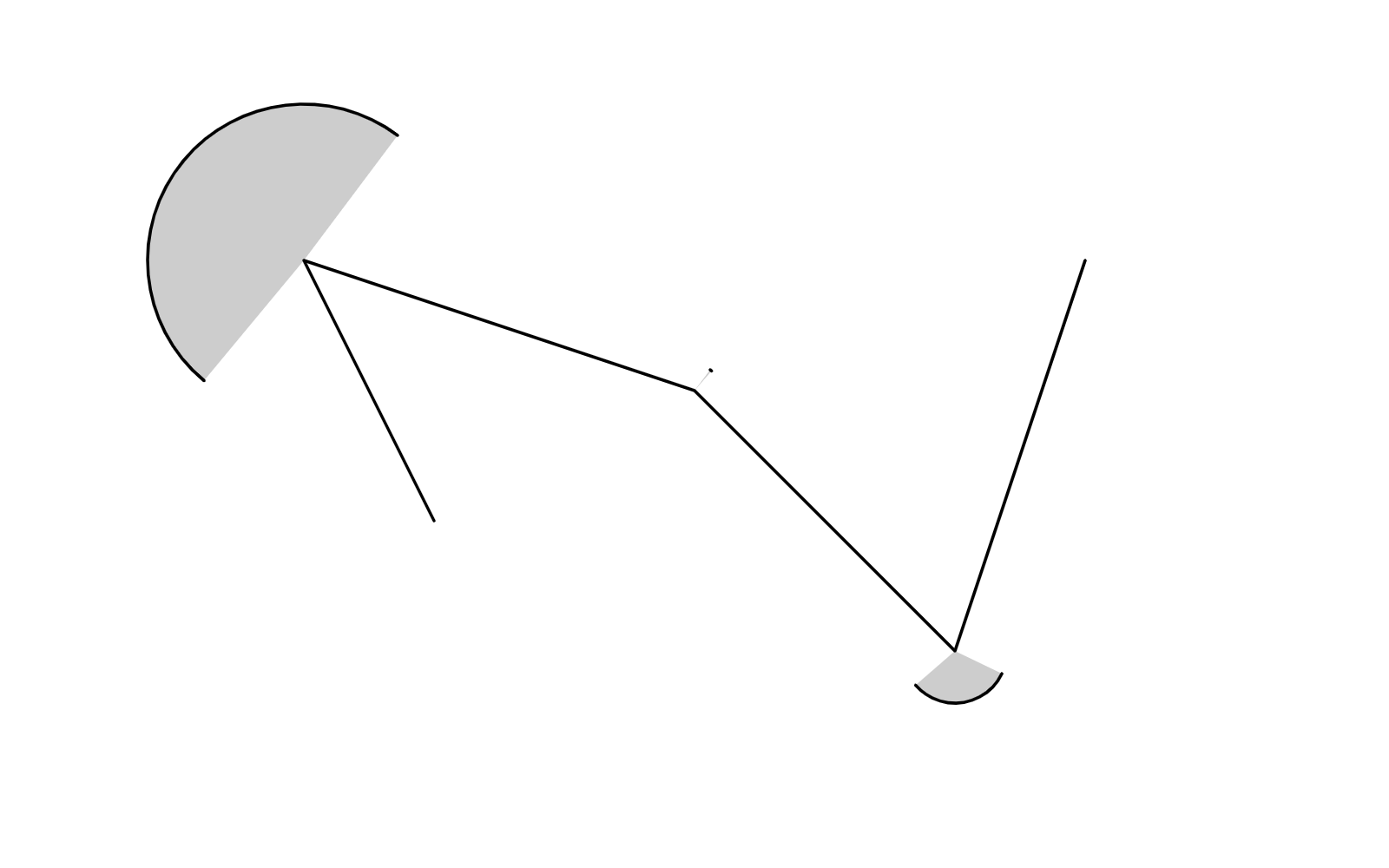Note that the arcs need to be clockwise (or whichever winding the rest of the outline is). This is especially important when sampling the arcs into tiny segments. Assuming radians increase clockwise (as is common in computer graphics), to interpolate from angle $a$ to angle $b$ clockwise:

function interpolate(a:number, b:number, t:number):number{
// 0 <= a <= 2*PI, 0 <= b <= 2*PI
// 0 <= t <= 1
if (a < b){
return a * (1-t) + b * t;
}else{
return a * (1-t) + (b + Math.PI * 2) * t;
}
}


But wait a moment, with the varying radii, things can get more complex, consider the following case where the middle circle is much smaller than its neighbors: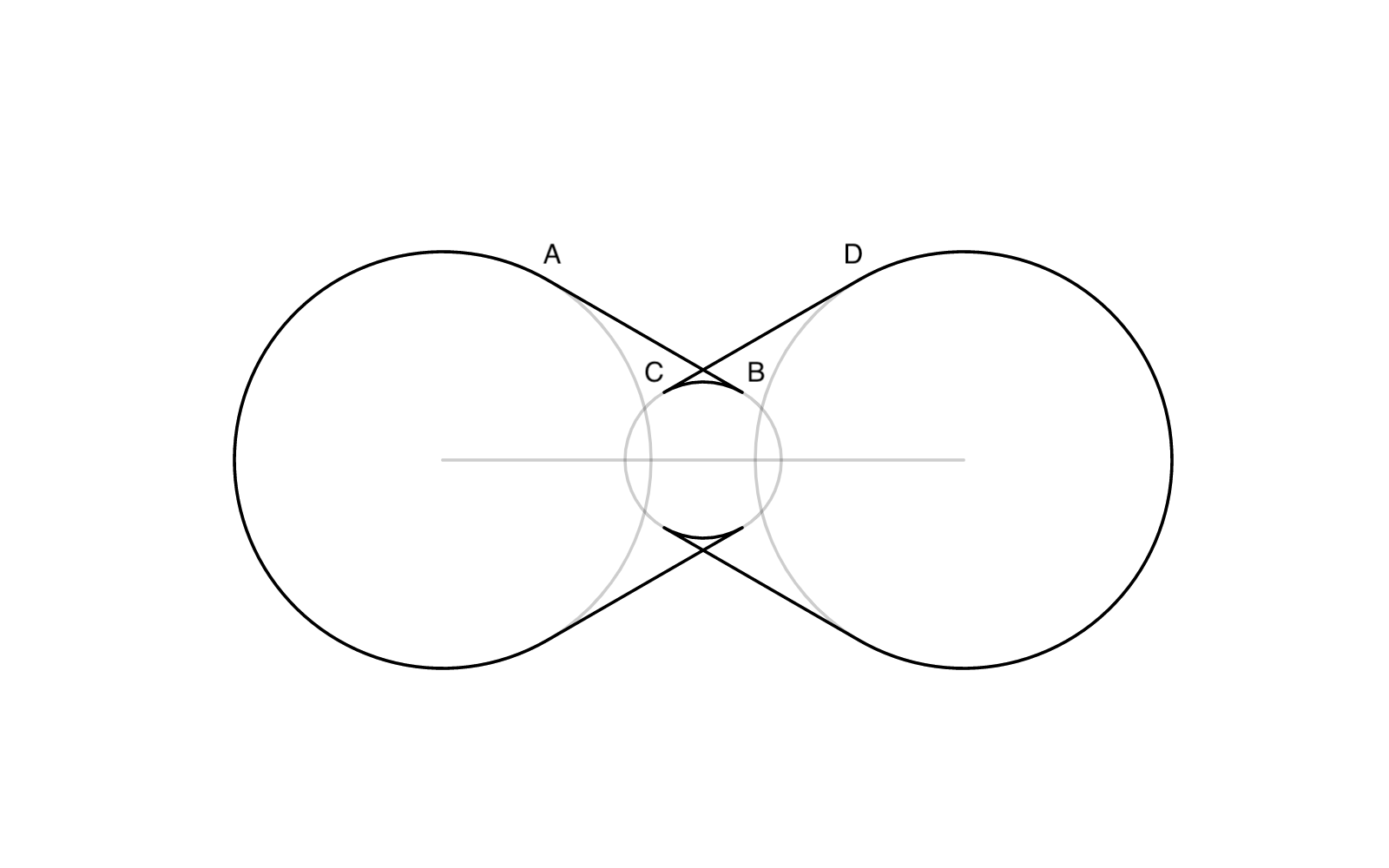Notice the reversed hoops. We either need to skip drawing the arc for either side of the vertex, or the arcs need to be counter-clockwise instead of clockwise.

Therefore, the more general rule is, if $AB$ intersects $CD$ (these are the segments we computed in the previous step for each edge), then we need to draw a clockwise arc, otherwise, we skip it.

When implementing this, I encountered additional degenerate cases. They're not those deadly ones, but they create redundant arcs that slows down later processing steps. So in the end, I found this heuristic which seems to work for all:

If polyline is turning right at the given vertex, define the LHS (left-hand-side) as the “major” side, and vice versa.

• If the LHS segments intersect:
• If LHS is major, draw the LHS arc clockwise;
• Otherwise, draw the LHS arc either clockwise or counter-clockwise, whichever is the shorter arc.
• If the RHS segments intersect:
• If RHS is major, draw the RHS arc clockwise;
• Otherwise, draw the RHS arc either clockwise or counter-clockwise, whichever is the shorter arc.

Finally, combining all the parts, we get this: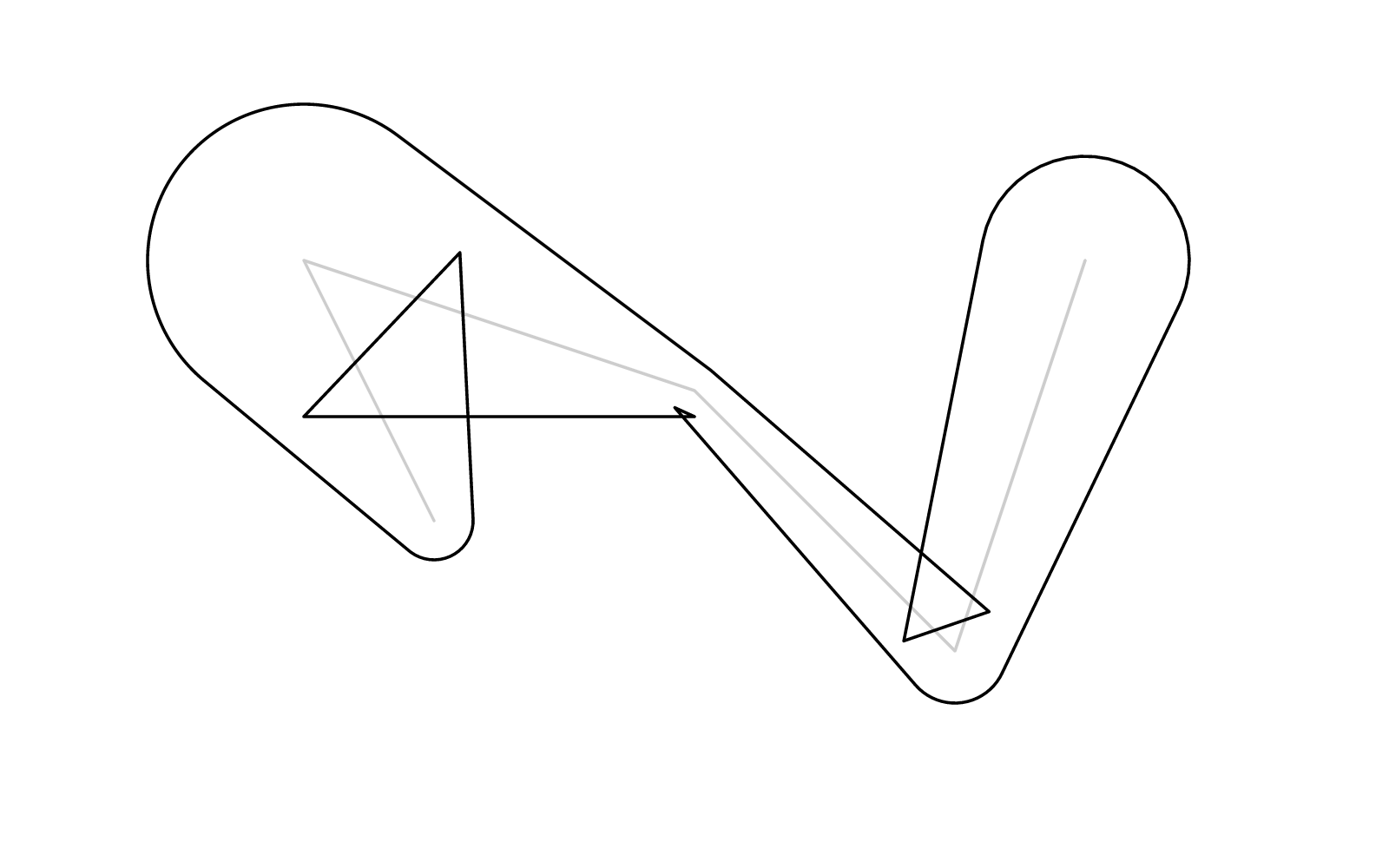There's still a bunch of self-intersections, which we want to get rid of. Luckily, in my previous post, I described exactly how to do that. There's also a demo.

Finally, we get the perfectly clean outline: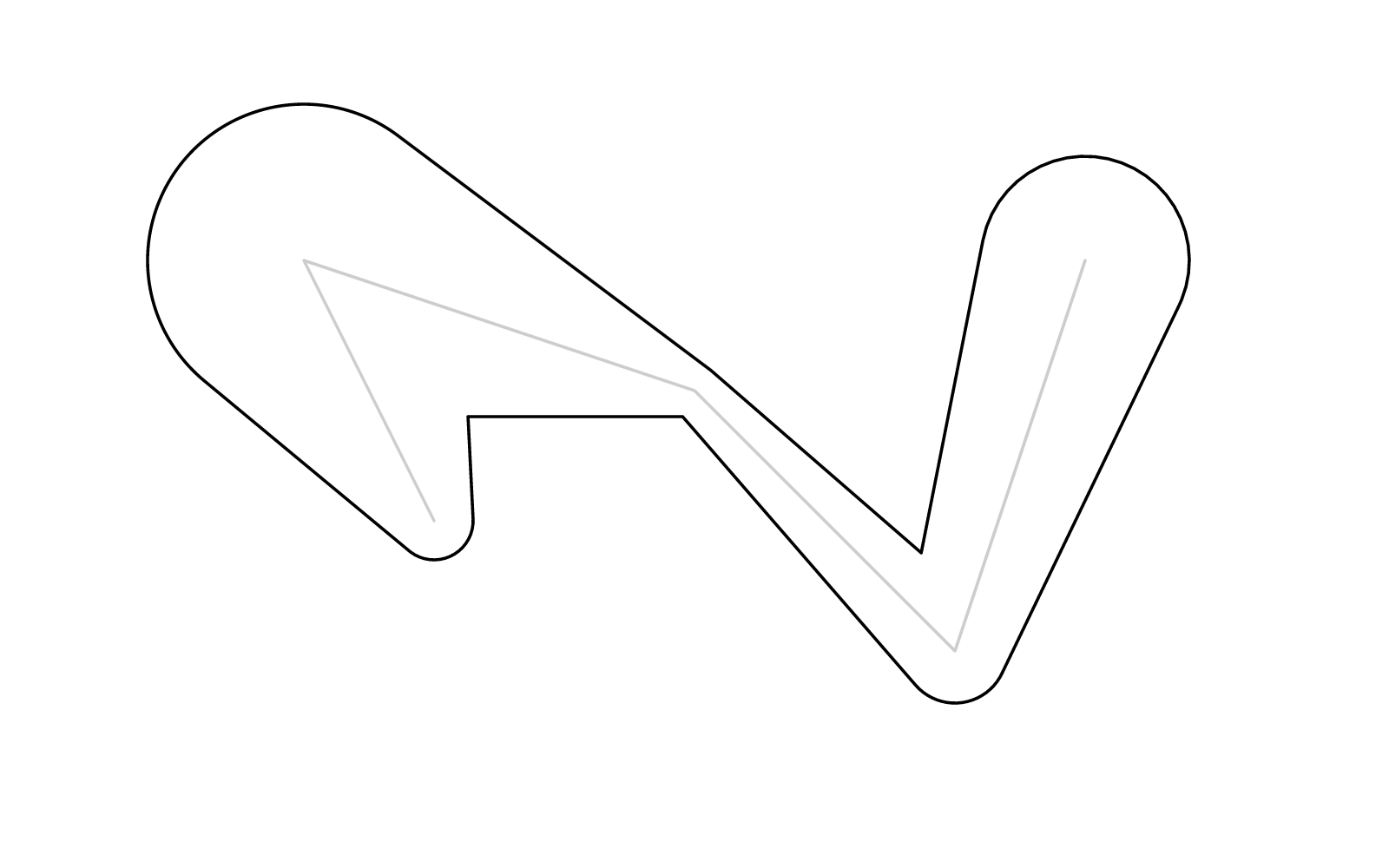## Miter

Besides “round” and “bevel” join styles we just saw, there's also what's known as the “miter”. It's easy enough to implement by replacing the arc with an angle bisector. However when the turn is too steep, the sharp corner will stab into infinity, and a “miter-limit” might be desirable.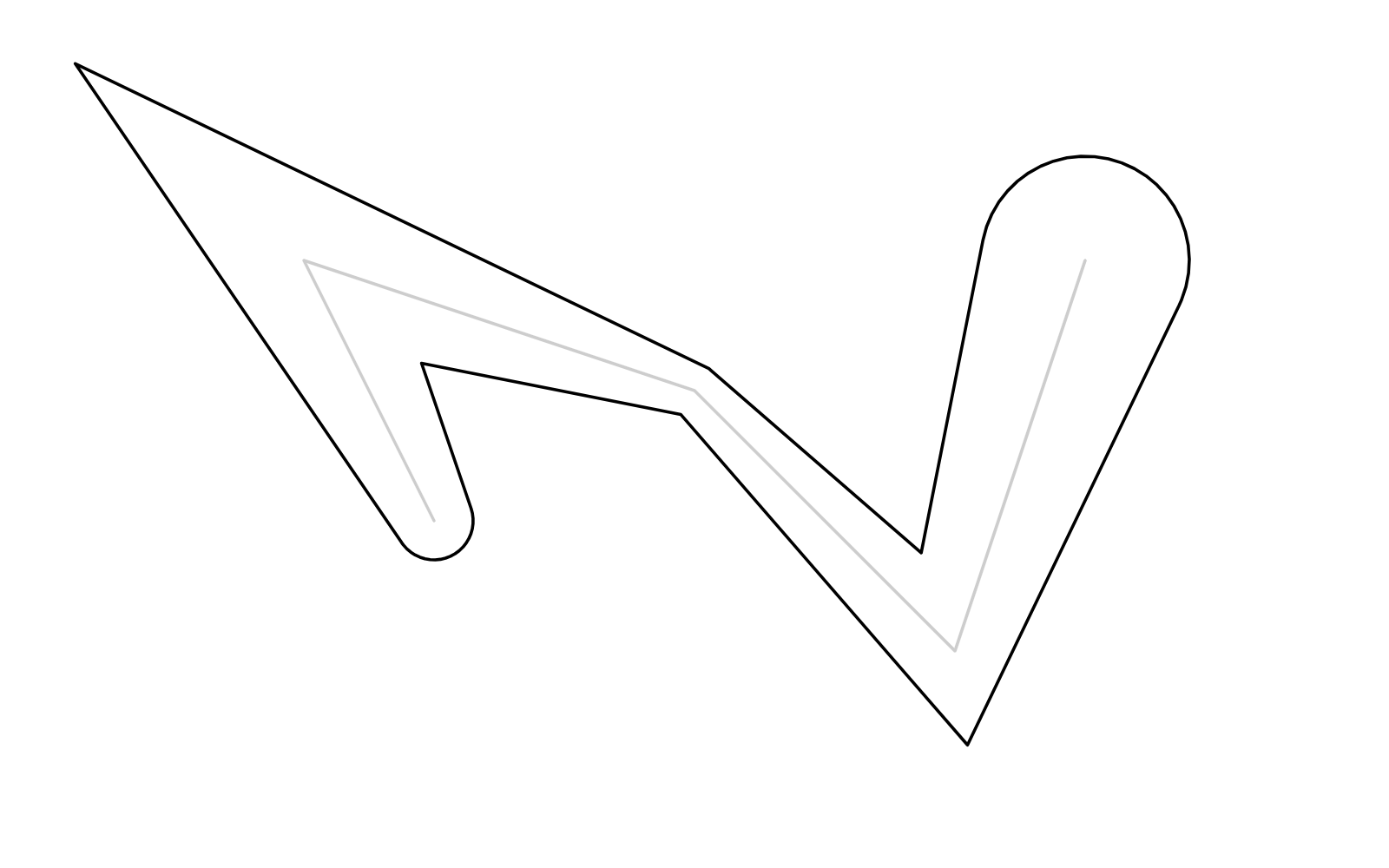## Degeneracy

But we're not done yet, there're some nasty degenerate cases. When a segment is too short, the circle of one vertex might entirely engulf that of the next, and there'll be no tangent.

Here's the critical situation right before it becomes degenerate: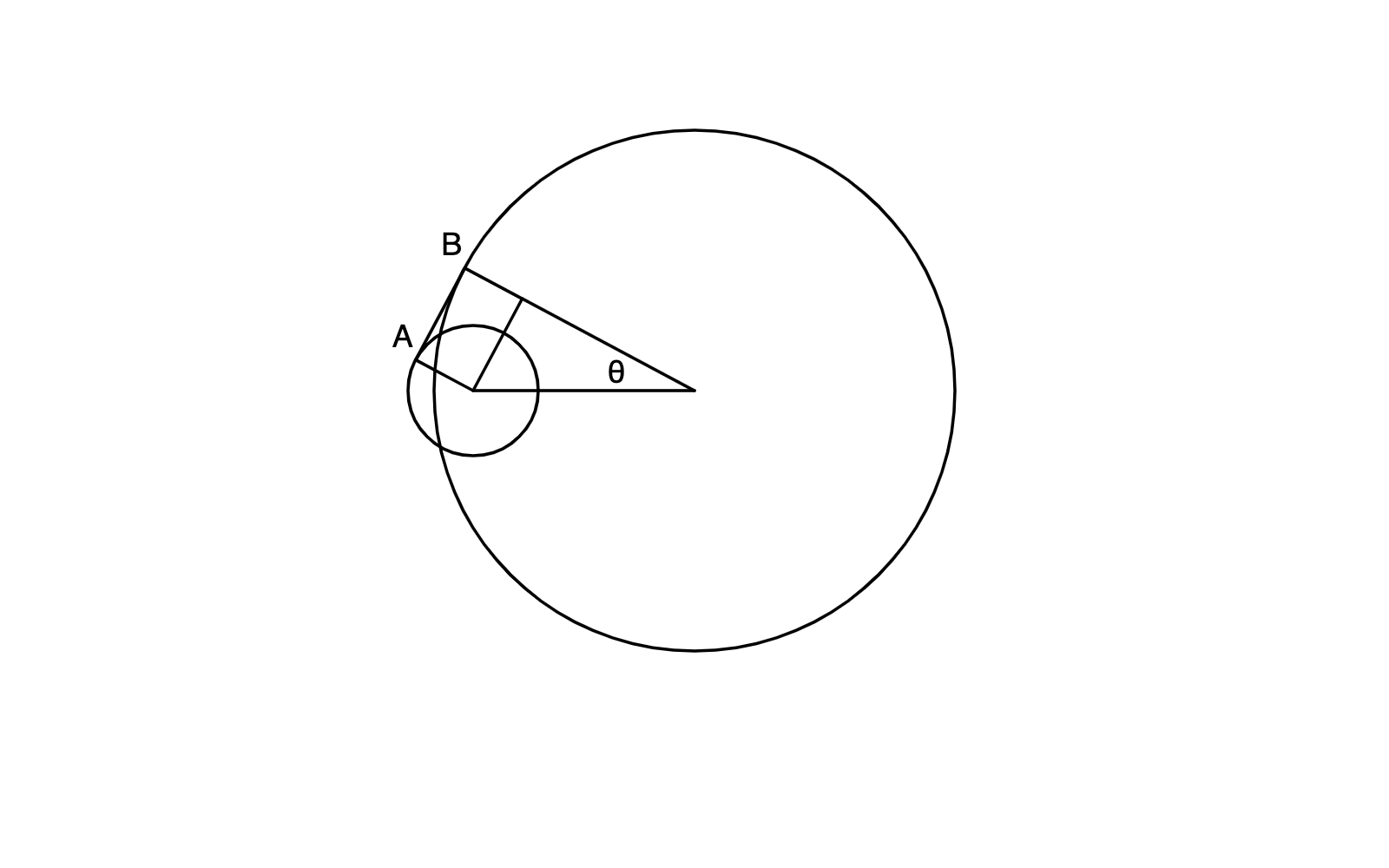Now it's totally degenerate, and $\theta$ and $AB$ are nowhere to be found: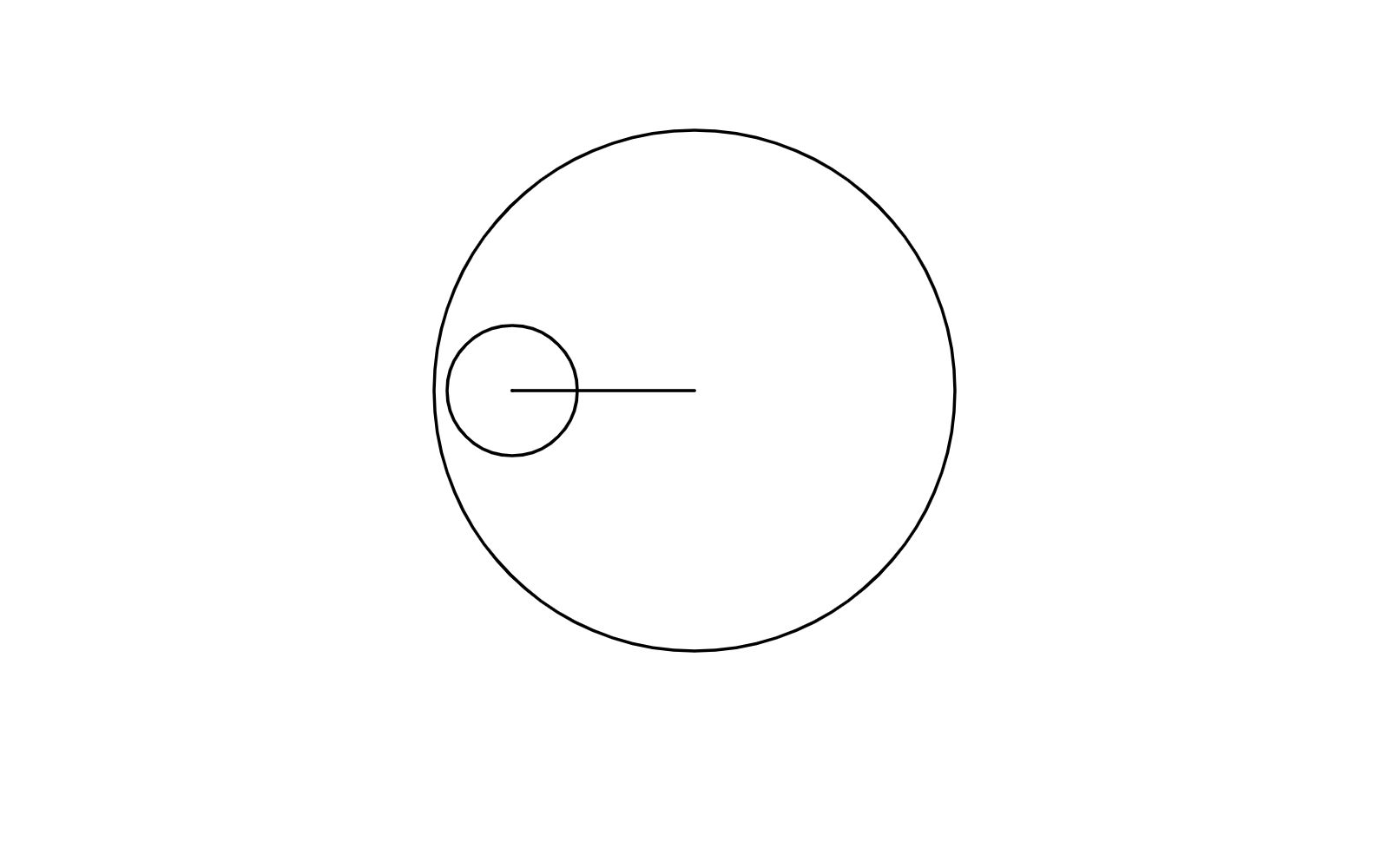You might think: well let's just get rid of the smaller circle! But there's a problem with that. Say we have this situation: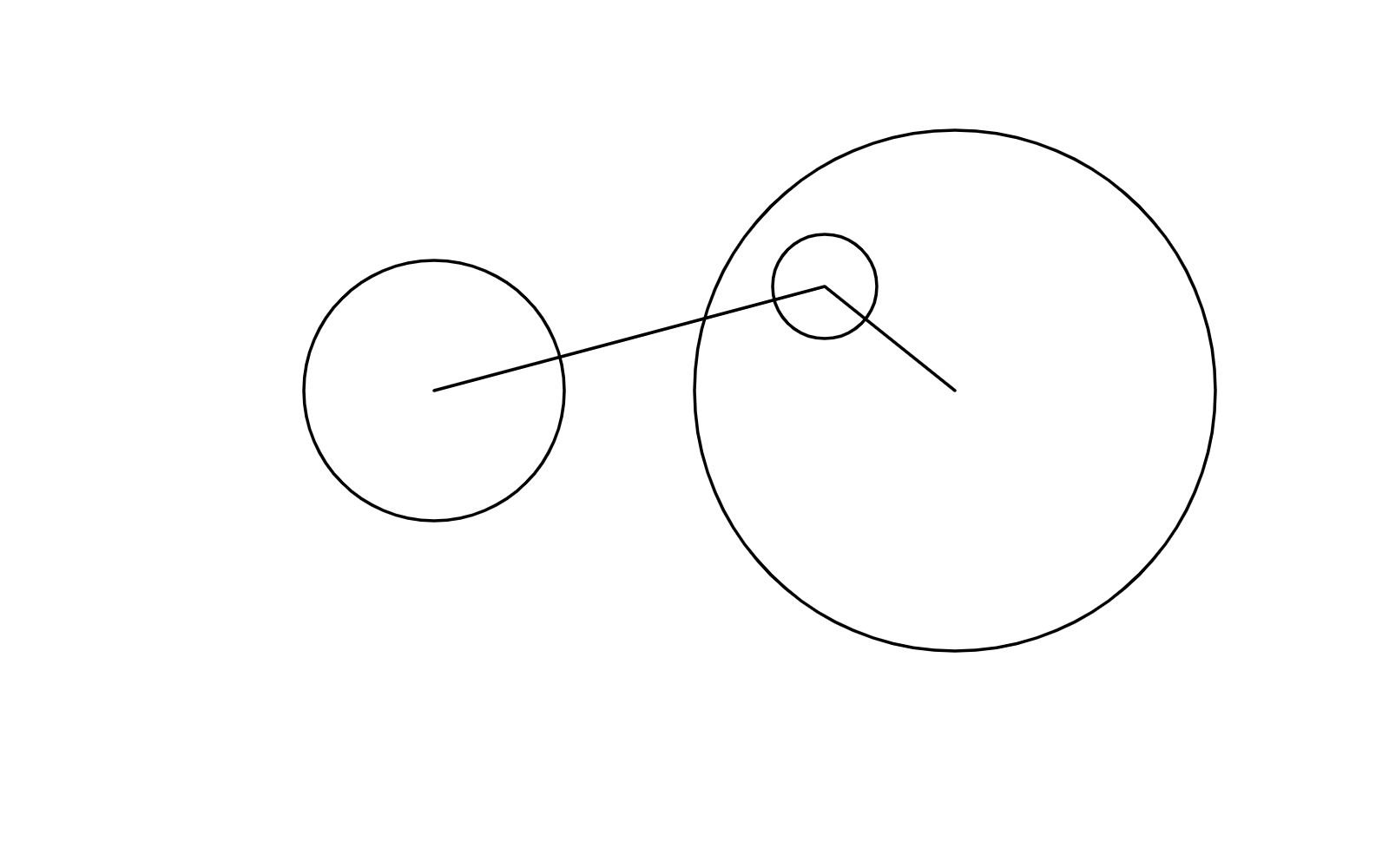If we just get rid of the small circle, we'll get this outline: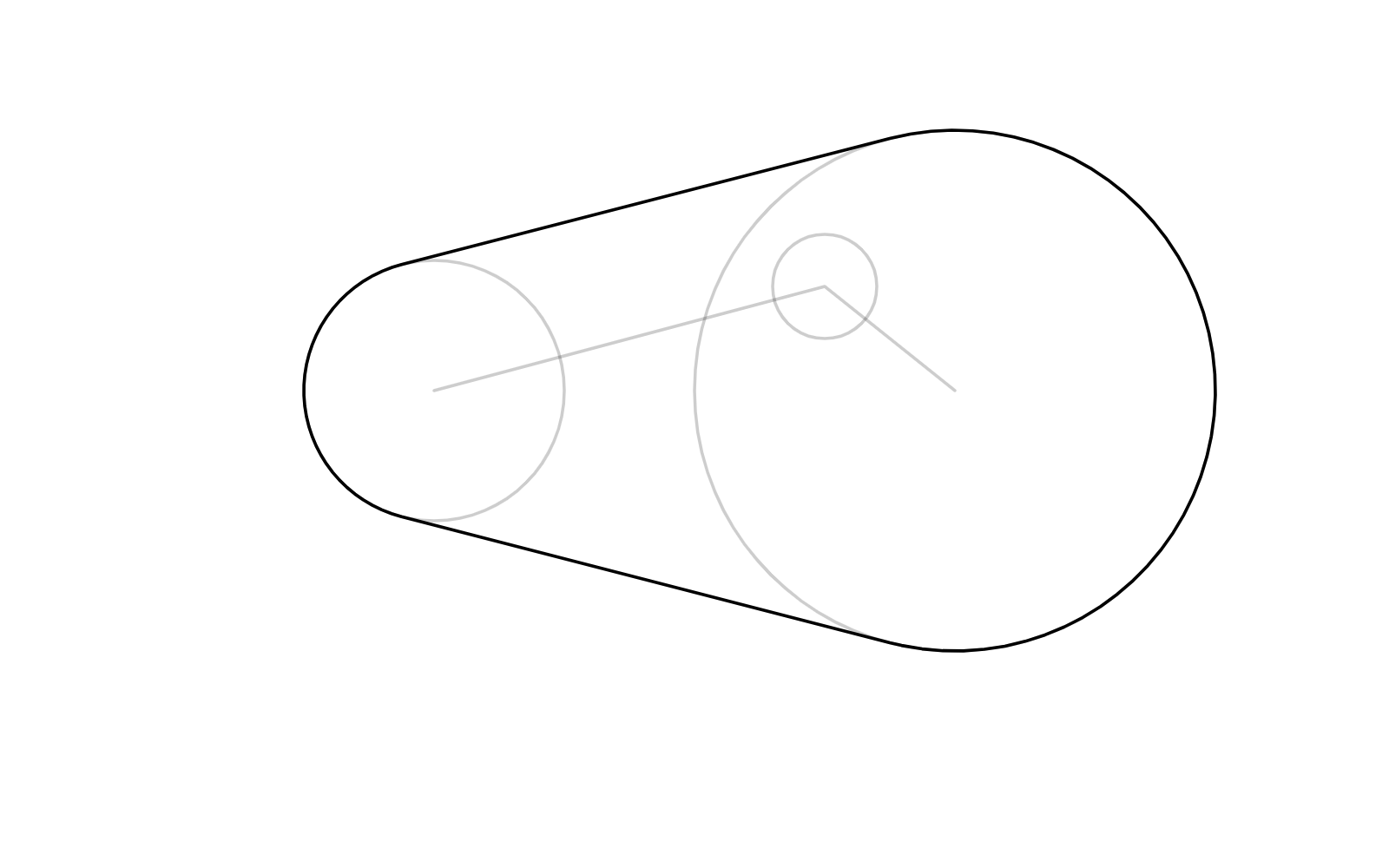However, what we might instead want something like this: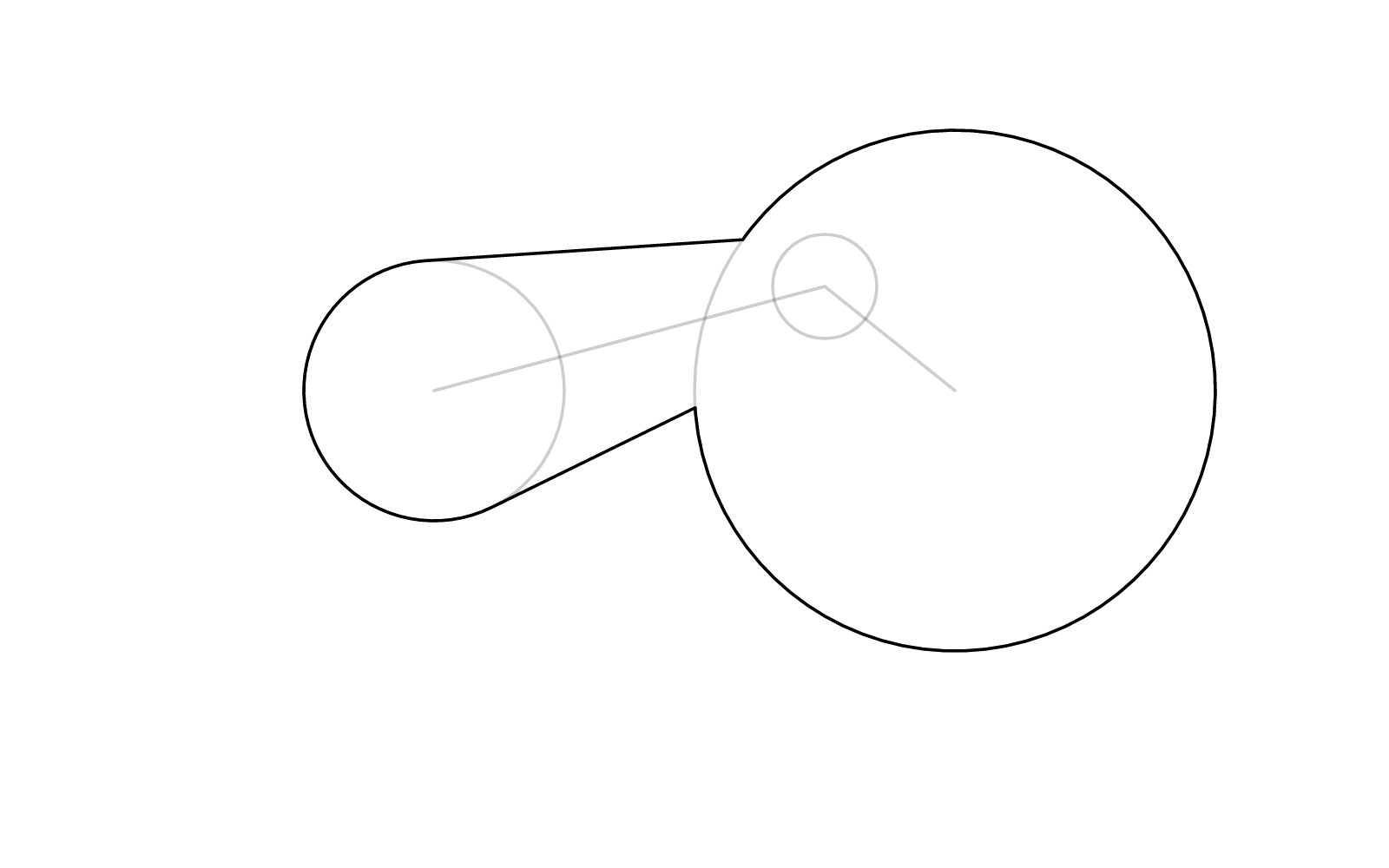One solution is, instead of removing any circle, generate “placeholder” bridges that take the place of the tangents, like so: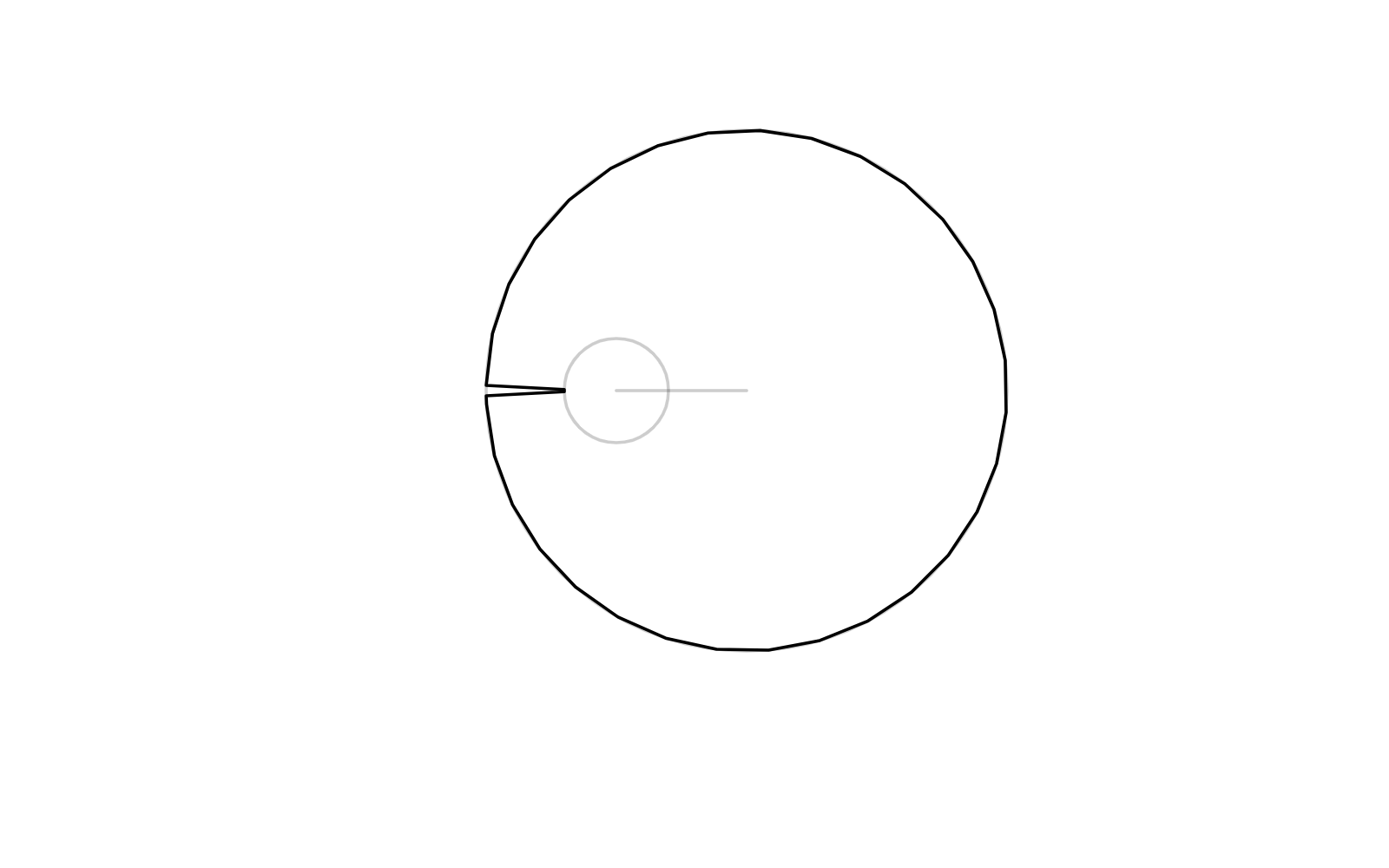This produces better behavior when further connecting the duo to a third vertex: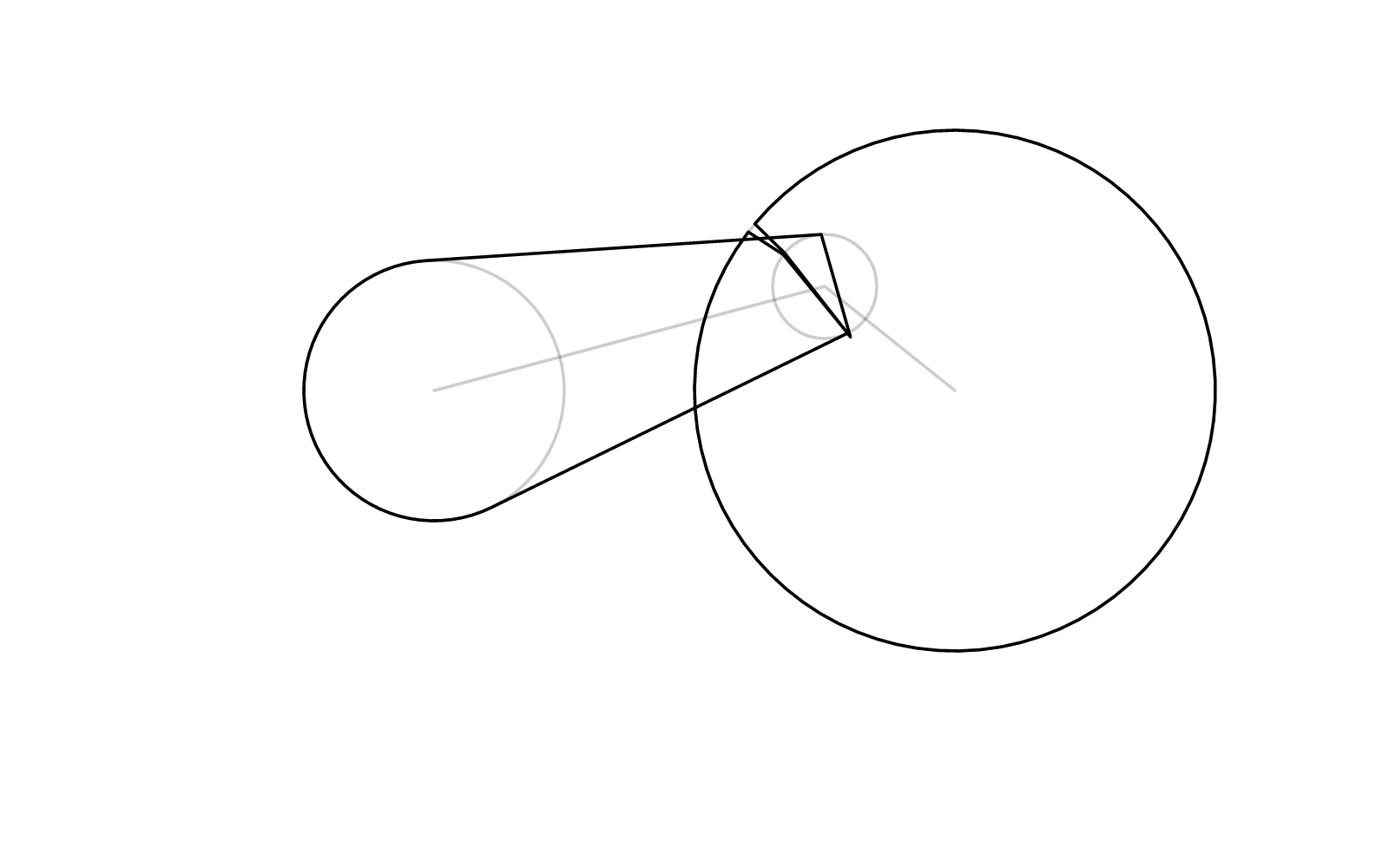Then we send this mess to our self-intersection-removal algirthm to sort out: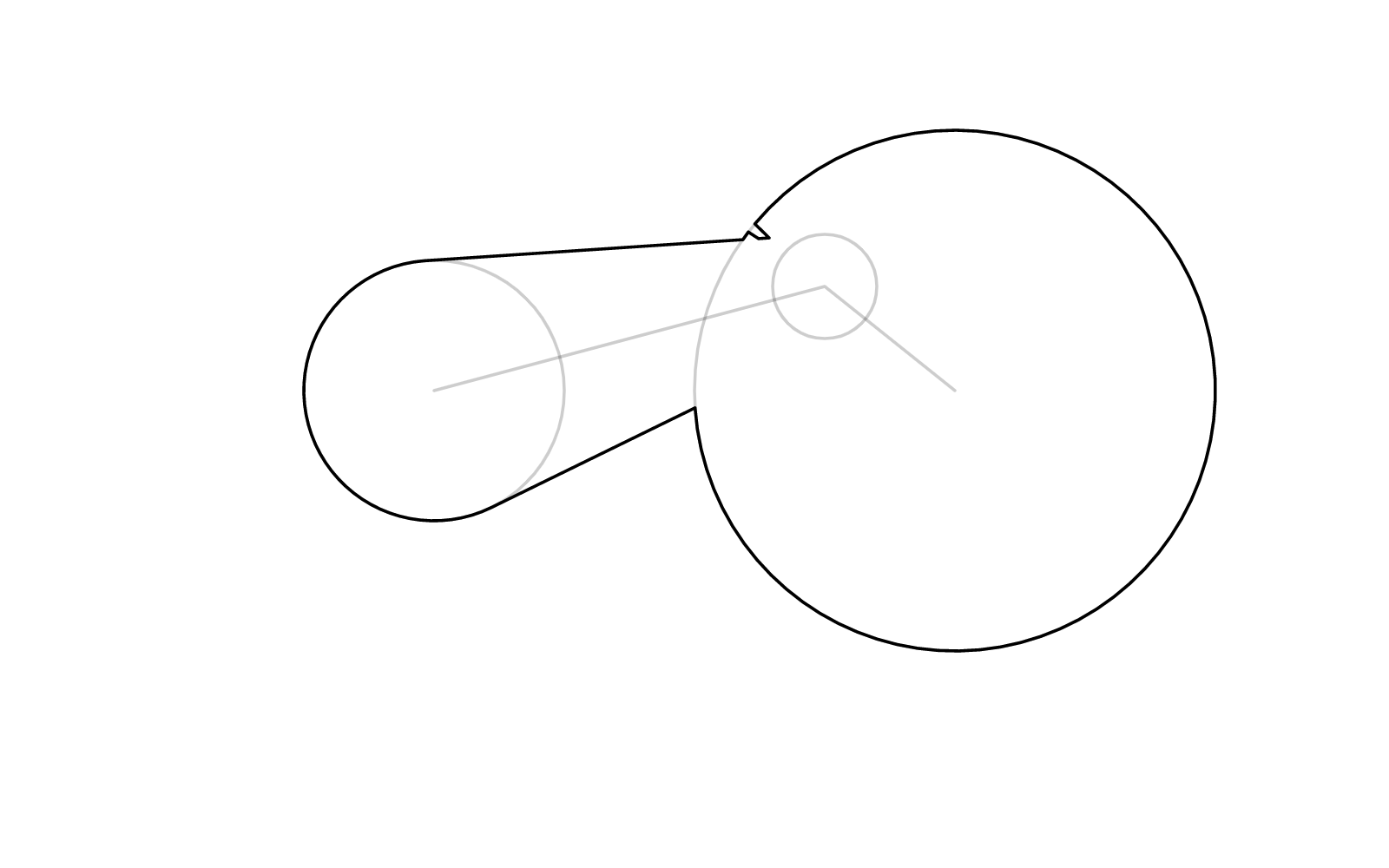As you can see above, a defect “incision point” might appear where we generated the bridge. However, we can either make the bridge too skinny to notice, or hide it by rotating it to a more “convenient” angle (depending where the third vertex is), and let the self-intersection-removal algorithm dispose of it. You get the idea.

## Code

Here's a TypeScript implementation of the hose-making algorithm described above. It is capable of round, miter, and bevel join styles, as well as round and flat caps. It takes in the polyline, a corresponding array of radii, and some more options.

This code is part of a vector brushstroke library I'm currently working on, and might receive updates and fixes as I improve the library.

This code does not include self-intersection removal. You can find that in my previous post instead.

export function cwise(p1x:number, p1y:number, p2x:number, p2y:number, p3x:number, p3y:number):number{
return (p2x - p1x)*(p3y - p1y) - (p2y - p1y)*(p3x - p1x)
}

export function interp_angles(a0:number,a1:number,step:number,dir:number=0,max:number=6):number[]{
a0 = (a0 + Math.PI*2)%(Math.PI*2);
a1 = (a1 + Math.PI*2)%(Math.PI*2);
function make_interval(a0:number,a1:number){
let o = [];
if (a0 < a1){
for (let a = a0+step; a < a1; a+= step){
o.push(a);
}
}else{
for (let a = a0-step; a > a1; a-= step){
o.push(a);
}
}
return o;
}
if (dir == undefined || dir == 0){
var methods : [number,Function][] = [
[Math.abs(a1-a0),           ()=>make_interval(a0,a1)],
[Math.abs(a1+Math.PI*2-a0), ()=>make_interval(a0,a1+Math.PI*2)],
[Math.abs(a1-Math.PI*2-a0), ()=>make_interval(a0,a1-Math.PI*2)]
]
methods.sort((x,y)=>(x-y))
return methods();
}else{
if (dir < 0){
while (a1 > a0){
a1 -= Math.PI*2;
}
}else{
while (a1 < a0){
a1 += Math.PI*2;
}
}
if (dir && Math.abs(a0-a1)>max){
return interp_angles(a0,a1,step,0);
}
return make_interval(a0,a1);
}
}

export function bisect_angles(a0:number,a1:number,dir:number=0,max:number=6):[number,number]{
a0 = (a0 + Math.PI*2)%(Math.PI*2);
a1 = (a1 + Math.PI*2)%(Math.PI*2);
function bisect(a0:number,a1:number):[number,number]{
return [(a0+a1)/2,Math.abs((a1-a0)/2)];
}
if (dir == undefined || dir == 0){
var methods : [number,Function][] = [
[Math.abs(a1-a0),           ()=>bisect(a0,a1)],
[Math.abs(a1+Math.PI*2-a0), ()=>bisect(a0,a1+Math.PI*2)],
[Math.abs(a1-Math.PI*2-a0), ()=>bisect(a0,a1-Math.PI*2)]
]
methods.sort((x,y)=>(x-y));
return methods();
}else{
if (dir < 0){
while (a1 > a0){
a1 -= Math.PI*2;
}
}else{
while (a1 < a0){
a1 += Math.PI*2;
}
}
if (dir && Math.abs(a0-a1)>max){
return bisect_angles(a0,a1,0);
}
return bisect(a0,a1);
}
}

function seg_isect(
p0x:number, p0y:number, p1x:number, p1y:number,
q0x:number, q0y:number, q1x:number, q1y:number) : boolean{

let d0x = ((p1x) - (p0x));
let d0y = ((p1y) - (p0y));
let d1x = ((q1x) - (q0x));
let d1y = ((q1y) - (q0y));
let vc = ((((d0x) * (d1y))) - (((d0y) * (d1x))));
if ((vc) == (0)) {
return false;
}
let vcn = ((vc) * (vc));
let q0x_p0x = (q0x) - (p0x);
let q0y_p0y = (q0y) - (p0y);
let vc_vcn = vc/vcn;
let t = ((((((((q0x_p0x)) * (d1y))) )) - ((((((q0y_p0y)) * (d1x))) )))) * vc_vcn;
let s = ((((((((q0x_p0x)) * (d0y))) )) - ((((((q0y_p0y)) * (d0x))) )))) * vc_vcn;
if (0 < t && t <= 1 && 0 <= s && s <= 1){
return true;
}
return false;
}

export function make_hose(polyline:[number,number][],widths:number[],join:string='round',cap:string="round",join_resolution:number=2,miter_limit:number=2){
let EPS = 0.001;
if (!polyline.length){
return [];
}
if (polyline.length < 2){
let p = polyline.slice();
p+=EPS;
polyline = polyline.concat([p as any]);
}
let angs : [number,number][] = [];
let lens : number[] = [];
for (let i = 0; i < polyline.length-1; i++){
let a = polyline[i];
let b = polyline[i+1];
let dx = b-a;
let dy = b-a;
let l = Math.sqrt(dx*dx+dy*dy);
let w0 = widths[i];
let w1 = widths[i+1];
let dw = w0 - w1;
let a0 = Math.atan2(dy,dx);

if (Math.abs(dw) < l-EPS){
let ang = Math.acos(dw/l);
angs.push([a0+ang,a0-ang]);
}else{
if (dw < 0){
angs.push([a0+Math.PI-EPS,a0-Math.PI+EPS]);
}else{
angs.push([a0+EPS,a0-EPS]);
}
}

lens.push(l);
}

let l0 : [number,number][] = [];
let l1 : [number,number][] = [];
for (let i = 0; i < polyline.length-1; i++){
let a = polyline[i];
let b = polyline[i+1];
let w0 = widths[i];
let w1 = widths[i+1];

let [a0,a1] = angs[i];

l0.push([a+Math.cos( a0)*w0,a+Math.sin( a0)*w0]);
l1.push([a+Math.cos( a1)*w0,a+Math.sin( a1)*w0]);
l0.push([b+Math.cos( a0)*w1,b+Math.sin( a0)*w1]);
l1.push([b+Math.cos( a1)*w1,b+Math.sin( a1)*w1]);

}

let j0 : [number,number][][] = [[]];
let j1 : [number,number][][] = [[]];
for (let i = 1; i < polyline.length-1; i++){
let a = polyline[i-1];
let b = polyline[i];
let c = polyline[i+1];
let major = cwise(...a,...b,...c)>0;
let do0 = true;
let do1 = true;
{
let p0 = l1[(i-1)*2];
let p1 = l1[(i-1)*2+1];
let p2 = l1[i*2];
let p3 = l1[i*2+1];

if (seg_isect(...p0,...p1,...p2,...p3)){
do0 = false;
}
}{
let p0 = l0[(i-1)*2];
let p1 = l0[(i-1)*2+1];
let p2 = l0[i*2];
let p3 = l0[i*2+1];

if (seg_isect(...p0,...p1,...p2,...p3)){
do1 = false;
}
}

if (join != 'bevel' && (do1 || do0)){
if (join != 'miter'){ //round
let step = Math.asin((join_resolution/2)/widths[i])*2;
if (isNaN(step)){
j1.push([]);
j0.push([]);
continue;
}

if (do0){
let a0 = angs[i-1];
let a1 = angs[i];

let jj = [];
let aa = interp_angles(a0,a1,step,major?1:0);
aa.forEach(a=>{
let dx = Math.cos(a)*widths[i];
let dy = Math.sin(a)*widths[i];
jj.push([b+dx,b+dy]);
})
j1.push(jj);
if (!do1)j0.push([]);

}
if (do1){
let a0 = angs[i-1];
let a1 = angs[i];
let jj = [];

let aa = interp_angles(a0,a1,step,major?0:-1);
aa.forEach(a=>{
let dx = Math.cos(a)*widths[i];
let dy = Math.sin(a)*widths[i];
jj.push([b+dx,b+dy]);
})
j0.push(jj);
if (!do0)j1.push([]);
}
}else{//miter

if (do0){
let a0 = angs[i-1];
let a1 = angs[i];

let [aa,ab] = bisect_angles(a0,a1,major?1:0);
let w = Math.abs(widths[i]/Math.cos(ab));
w = Math.min(widths[i]*miter_limit,w);
let jj : [number,number][] = [[b+w*Math.cos(aa),b+w*Math.sin(aa)]]
j1.push(jj);
if(!do1)j0.push([]);

}
if (do1){
let a0 = angs[i-1];
let a1 = angs[i];

let [aa,ab] = bisect_angles(a0,a1,major?0:-1);
let w = Math.abs(widths[i]/Math.cos(ab));
w = Math.min(widths[i]*miter_limit,w);
let jj : [number,number][] = [[b+w*Math.cos(aa),b+w*Math.sin(aa)]]

j0.push(jj);
if (!do0)j1.push([]);
}
}
}else{
j0.push([]);
j1.push([]);
}
}
let ll0 : [number,number][]= [];
let ll1 : [number,number][]= [];
for (let i = 0; i < l0.length/2; i++){
ll0.push(...j0[i]);
ll1.push(...j1[i]);

ll0.push(l0[i*2]);
ll0.push(l0[i*2+1]);
ll1.push(l1[i*2]);
ll1.push(l1[i*2+1]);

}
l0 = ll0;
l1 = ll1;
if (cap == 'round'){{
let jj = [];
let a = polyline;
let b = polyline;
let [a0,a1] = angs;
let step = Math.asin((join_resolution/2)/widths)*2;
let aa = interp_angles(a0,a1,step,1);
if (!isNaN(step)){
aa.forEach(z=>{
let x = a + widths*Math.cos(z);
let y = a + widths*Math.sin(z);
jj.push([x,y]);
});
}
l1 = jj.concat(l1);
}{
let jj : [number,number][] = [];
let a = polyline[polyline.length-2];
let b = polyline[polyline.length-1];
let [a1,a0] = angs[polyline.length-2];

let step = Math.asin((join_resolution/2)/widths)*2;
let aa = interp_angles(a0,a1,step,1);
if (!isNaN(step)){
aa.forEach(z=>{
let x = b + widths[widths.length-1]*Math.cos(z);
let y = b + widths[widths.length-1]*Math.sin(z);
jj.push([x,y]);
});
}
l1.push(...jj);
}}

l0.reverse();
let ret = l1.concat(l0);
return ret;
}